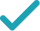Type a math problemSolve for bSteps Using the Quadratic Formula
Steps for Completing the Square
Variable cannot be equal to any of the values since division by zero is not defined. Multiply both sides of the equation by , the least common multiple of .
Use the distributive property to multiply by .
Use the distributive property to multiply by .
To find the opposite of , find the opposite of each term.
Combine and to get .
Use the distributive property to multiply by .
Use the distributive property to multiply by and combine like terms.
Subtract from both sides.
Combine and to get .
All equations of the form can be solved using the quadratic formula: . The quadratic formula gives two solutions, one when is addition and one when it is subtraction.
This equation is in standard form: . Substitute for , for , and for in the quadratic formula, .
Square .
Multiply times .
Multiply times .
Take the square root of .
Multiply times .
Now solve the equation when is plus. Add to .
Divide by .
Now solve the equation when is minus. Subtract from .
Divide by .
The equation is now solved.
\left(2b+1\right)\times 2-\left(b-3\right)\times 6=4\left(b-3\right)\left(2b+1\right)
Variable b cannot be equal to any of the values -\frac{1}{2},3 since division by zero is not defined. Multiply both sides of the equation by \left(b-3\right)\left(2b+1\right), the least common multiple of b-3,2b+1.
4b+2-\left(b-3\right)\times 6=4\left(b-3\right)\left(2b+1\right)
Use the distributive property to multiply 2b+1 by 2.
4b+2-\left(6b-18\right)=4\left(b-3\right)\left(2b+1\right)
Use the distributive property to multiply b-3 by 6.
4b+2-6b+18=4\left(b-3\right)\left(2b+1\right)
To find the opposite of 6b-18, find the opposite of each term.
-2b+2+18=4\left(b-3\right)\left(2b+1\right)
Combine 4b and -6b to get -2b.
-2b+20=4\left(b-3\right)\left(2b+1\right)
Add 2 and 18 to get 20.
-2b+20=\left(4b-12\right)\left(2b+1\right)
Use the distributive property to multiply 4 by b-3.
-2b+20=8b^{2}-20b-12
Use the distributive property to multiply 4b-12 by 2b+1 and combine like terms.
-2b+20-8b^{2}=-20b-12
Subtract 8b^{2} from both sides.
-2b+20-8b^{2}+20b=-12
18b+20-8b^{2}=-12
Combine -2b and 20b to get 18b.
18b+20-8b^{2}+12=0
18b+32-8b^{2}=0
Add 20 and 12 to get 32.
-8b^{2}+18b+32=0
All equations of the form ax^{2}+bx+c=0 can be solved using the quadratic formula: \frac{-b±\sqrt{b^{2}-4ac}}{2a}. The quadratic formula gives two solutions, one when ± is addition and one when it is subtraction.
b=\frac{-18±\sqrt{18^{2}-4\left(-8\right)\times 32}}{2\left(-8\right)}
This equation is in standard form: ax^{2}+bx+c=0. Substitute -8 for a, 18 for b, and 32 for c in the quadratic formula, \frac{-b±\sqrt{b^{2}-4ac}}{2a}.
b=\frac{-18±\sqrt{324-4\left(-8\right)\times 32}}{2\left(-8\right)}
Square 18.
b=\frac{-18±\sqrt{324+32\times 32}}{2\left(-8\right)}
Multiply -4 times -8.
b=\frac{-18±\sqrt{324+1024}}{2\left(-8\right)}
Multiply 32 times 32.
b=\frac{-18±\sqrt{1348}}{2\left(-8\right)}
b=\frac{-18±2\sqrt{337}}{2\left(-8\right)}
Take the square root of 1348.
b=\frac{-18±2\sqrt{337}}{-16}
Multiply 2 times -8.
b=\frac{2\sqrt{337}-18}{-16}
Now solve the equation b=\frac{-18±2\sqrt{337}}{-16} when ± is plus. Add -18 to 2\sqrt{337}\approx 36.715119501.
b=\frac{9-\sqrt{337}}{8}
Divide -18+2\sqrt{337}\approx 18.715119501 by -16.
b=\frac{-2\sqrt{337}-18}{-16}
Now solve the equation b=\frac{-18±2\sqrt{337}}{-16} when ± is minus. Subtract 2\sqrt{337}\approx 36.715119501 from -18.
b=\frac{\sqrt{337}+9}{8}
Divide -18-2\sqrt{337}\approx -54.715119501 by -16.
b=\frac{9-\sqrt{337}}{8} b=\frac{\sqrt{337}+9}{8}
The equation is now solved.
\left(2b+1\right)\times 2-\left(b-3\right)\times 6=4\left(b-3\right)\left(2b+1\right)
Variable b cannot be equal to any of the values -\frac{1}{2},3 since division by zero is not defined. Multiply both sides of the equation by \left(b-3\right)\left(2b+1\right), the least common multiple of b-3,2b+1.
4b+2-\left(b-3\right)\times 6=4\left(b-3\right)\left(2b+1\right)
Use the distributive property to multiply 2b+1 by 2.
4b+2-\left(6b-18\right)=4\left(b-3\right)\left(2b+1\right)
Use the distributive property to multiply b-3 by 6.
4b+2-6b+18=4\left(b-3\right)\left(2b+1\right)
To find the opposite of 6b-18, find the opposite of each term.
-2b+2+18=4\left(b-3\right)\left(2b+1\right)
Combine 4b and -6b to get -2b.
-2b+20=4\left(b-3\right)\left(2b+1\right)
Add 2 and 18 to get 20.
-2b+20=\left(4b-12\right)\left(2b+1\right)
Use the distributive property to multiply 4 by b-3.
-2b+20=8b^{2}-20b-12
Use the distributive property to multiply 4b-12 by 2b+1 and combine like terms.
-2b+20-8b^{2}=-20b-12
Subtract 8b^{2} from both sides.
-2b+20-8b^{2}+20b=-12
18b+20-8b^{2}=-12
Combine -2b and 20b to get 18b.
18b-8b^{2}=-12-20
Subtract 20 from both sides.
18b-8b^{2}=-32
Subtract 20 from -12 to get -32.
-8b^{2}+18b=-32
Quadratic equations such as this one can be solved by completing the square. In order to complete the square, the equation must first be in the form x^{2}+bx=c.
\frac{-8b^{2}+18b}{-8}=\frac{-32}{-8}
Divide both sides by -8.
b^{2}+\frac{18}{-8}b=\frac{-32}{-8}
Dividing by -8 undoes the multiplication by -8.
b^{2}-\frac{9}{4}b=\frac{-32}{-8}
Reduce the fraction \frac{18}{-8}=-2.25 to lowest terms by extracting and canceling out 2.
b^{2}-\frac{9}{4}b=4
Divide -32 by -8.
b^{2}-\frac{9}{4}b+\left(-\frac{9}{8}\right)^{2}=4+\left(-\frac{9}{8}\right)^{2}
Divide -\frac{9}{4}=-2.25, the coefficient of the x term, by 2 to get -\frac{9}{8}=-1.125. Then add the square of -\frac{9}{8}=-1.125 to both sides of the equation. This step makes the left hand side of the equation a perfect square.
b^{2}-\frac{9}{4}b+\frac{81}{64}=4+\frac{81}{64}
Square -\frac{9}{8}=-1.125 by squaring both the numerator and the denominator of the fraction.
b^{2}-\frac{9}{4}b+\frac{81}{64}=\frac{337}{64}